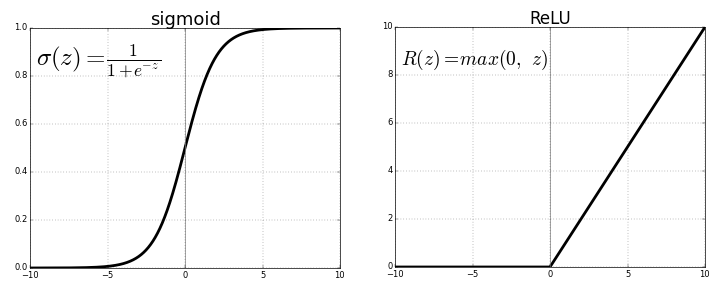## Introduction to Machine Learning#### Linear Regression

##### Linear Regression Data##### Linear Regression Visualization##### Linear Regression model
$\hspace{2.5cm} X \hspace{1.1cm} \times \hspace{1.2cm} W^T \hspace{1.2cm} + \hspace{1cm} b \hspace{2cm}$
$\left[ \begin{array}{cc} 73 & 67 & 43 \\ 91 & 88 & 64 \\ \vdots & \vdots & \vdots \\ 69 & 96 & 70 \end{array} \right] % \times % \left[ \begin{array}{cc} w_{11} & w_{21} \\ w_{12} & w_{22} \\ w_{13} & w_{23} \end{array} \right] % + % \left[ \begin{array}{cc} b_{1} & b_{2} \\ b_{1} & b_{2} \\ \vdots & \vdots \\ b_{1} & b_{2} \\ \end{array} \right]$

#### Feedfoward Neural NetworkConceptually, you think of feedforward neural networks as two or more linear regression models stacked on top of one another with a non-linear activation function applied between them.To use a feedforward neural network instead of linear regression, we can extend the nn.Module class from PyTorch.

!pip install jovian --upgrade -q
import jovian